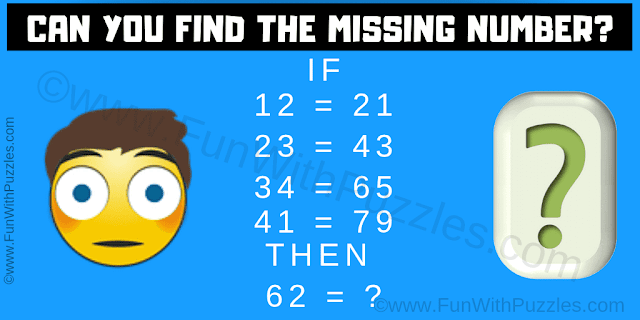This is the logical thinking puzzle test question which will challenge your mind. In this logical puzzle, you are shown some logical number equations. Your challenge is to decode the logical reasoning in these equations and then find the value of the missing number which will replace the question mark.Can you solve this Logical Thinking Puzzle?
The answer to this "Logical Thinking Puzzle Test Question", can be viewed by clicking on the answer button.